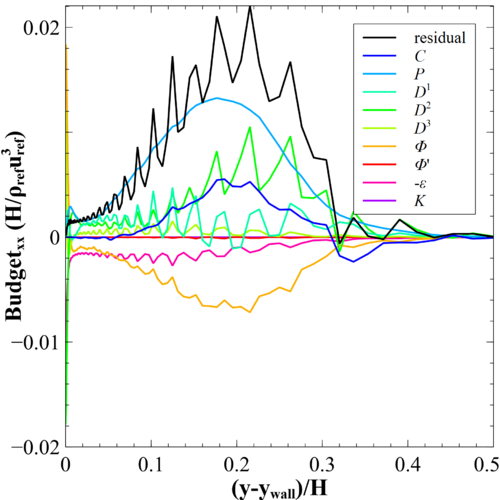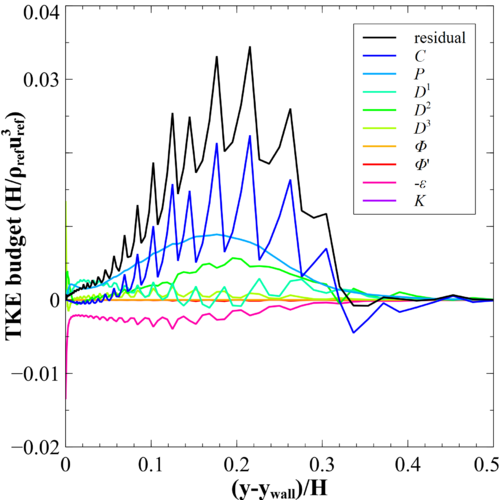# Quantification of resolution

This section provides details of the solution accuracy obtained by tackling the rounded step DNS with MIGALE. After providing details of the mesh resolution in comparison with spatial turbulent scales, a discussion on the closure of the Reynolds stress equations budgets is given.

## Mesh resolution

The mesh resolution is quantified by comparing the mesh characteristic length (${\Delta }$) with the characteristic lengths of the turbulence, i.e., the Taylor microscale (${\eta _{T}}$) and Kolmogorov length scale (${\eta _{K}}$). Here, the mesh characteristic length takes into account the degree of the DG polynomial approximation. In particular, it is defined as the cubic root of the ratio between the mesh element volume $\left(V\right)$and the number of DoFs $\left(N_{DoF}\right)$within the mesh element per equation

$\Delta ={\sqrt[{3}]{\dfrac {V}{N_{DoF}}}}$The comparison with respect to the Taylor microscale is shown in Fig. 6. The maximum ratio ${\Delta }/{\eta _{T}}$within the outer layer of the boundary layer is approximately 0.4. This outcome suggests that the current space resolution is sufficient to capture turbulence scales in the intertial range. In Fig. 7 is reported the comparison with respect to the Kolmogorov length scale. It is commonly accepted that DNS requirements are achieved when ${\Delta }/{\eta _{K}}\leq 5$. Current simulation shows above the flat plate upstream the rounded step a ratio ${\Delta }/{\eta _{K}}$below 5.5, while above the rounded step a ratio lower than 7.5. DNS requirements are thus not fulfilled in this last region. This is the reason why the present study is referred to as under-resolved DNS (uDNS). For future highly resolved simulations of this test case a mesh refinement is advised above and downstream the rounded step.Figure 6: HiFi-TURB-DLR rounded step, Re=78,490. Relation between the mesh size and the Taylor microscale at midspan using MIGALE with DG P3 (~300 million DoF/eqn).Figure 7: HiFi-TURB-DLR rounded step, Re=78,490. Relation between the mesh size and the Kolmogorov length scale at midspan using MIGALE with DG P3 (~300 million DoF/eqn).

The average wall resolution in streamwise (${\Delta x^{+}}$), spanwise (${\Delta z^{+}}$) and wall-normal (${\Delta y^{+}}$) directions at different streamwise locations is reported in Tab. 2.

 ${x/H}$${\Delta x^{+}}$${\Delta z^{+}}$${\Delta y_{1}^{+}}$${-3.5}$${17.8}$${18.6}$${1.30}$${3}$${6.53}$${8.19}$${0.55}$${4}$${3.90}$${5.71}$${0.40}$${5}$${9.22}$${9.28}$${0.66}$${6}$${12.8}$${13.0}$${0.93}$Table 2: Wall space resolution at different streamwise locations

## Solution verification

One way to verify that the DNS are properly resolved is to examine the budget of the Reynolds-stress equations and the turbulent kinetic energy (TKE) equation.

As first step, an assessment of code MIGALE in closing the budgets is performed. Fig. 8 reports the budget of streamwise Reynolds-stress and TKE equations in a channel flow at ${Re_{\tau }=180}$using a DG polynomial approximation of degree 5 on a mesh of $91\times 43\times 48$hexahedral elements (10.5 million DoF/eqn.). Domain dimension and reference results are given by DNS of Moser et al. (1999). As the maximum value of the residual is $0.22\%$and $0.64\%$of the production peak for the Reynolds-stress xx and the TKE budgets, respectively, the results show that MIGALE code can close well the budgets when sufficient spatial and time resolution is considered.

 Figure 8: Channel flow at $Re_{\tau }=180$Reynolds-stress xx and TKE budgets: dissipation $\varepsilon$, production $P$, turbulent diffusion $D^{1}$, pressure diffusion $D^{2}$, viscous diffusion $D^{3}$and pressure strain $\Phi$. Solid lines from the DG P5 computation and symbols from Moser et al. (1999).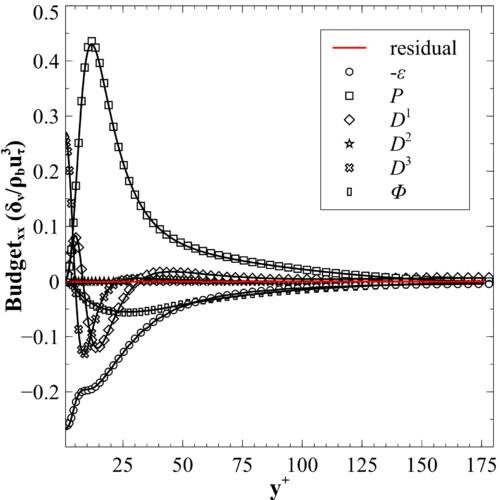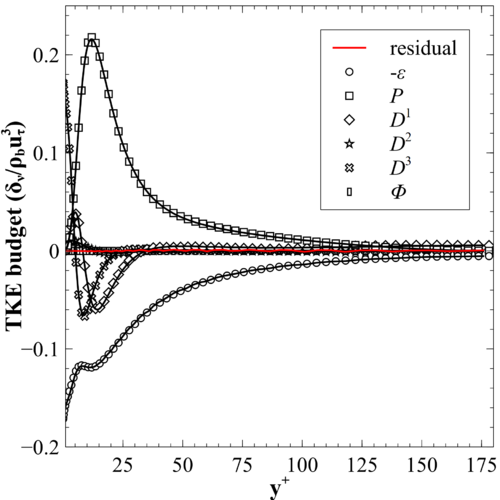Considering now the budgets for the HiFi-TURB-DLR rounded step simulation, the conclusion obtained comparing the mesh characteristic length and the Kolmogorov length scale (see Fig. 7) still holds. Indeed, on Fig. 9 and Fig. 10 are reported the budgets for two different steamwise locations, i.e., $x/H=-3$(near the checkpoint) and $x/H=4$(above the rounded step, in the region of incipient separation), respectively. As can be seen, budget closure has not been achieved, especially above the rounded step, where the residual is larger than the production term. The oscillating behaviour of the profiles of the different terms can be ascribed to a lack of spatial resolution. This result suggests that for future campaigns it is recommended to increase further the grid density.

 Figure 9: HiFi-TURB-DLR rounded step, Reynolds-stress xx and TKE budgets at location $x/H=-3$: convection $C$, production $P$, turbulent diffusion $D^{1}$, pressure diffusion $D^{2}$, viscous diffusion $D^{3}$, pressure strain $\Phi$, pressure dilatation $\Phi '$, dissipation $\varepsilon$and density fluctuation $K$.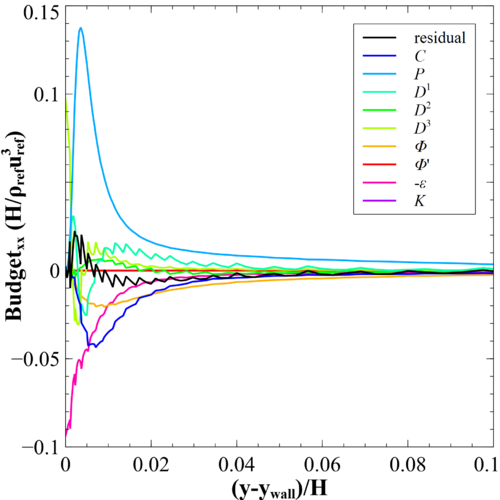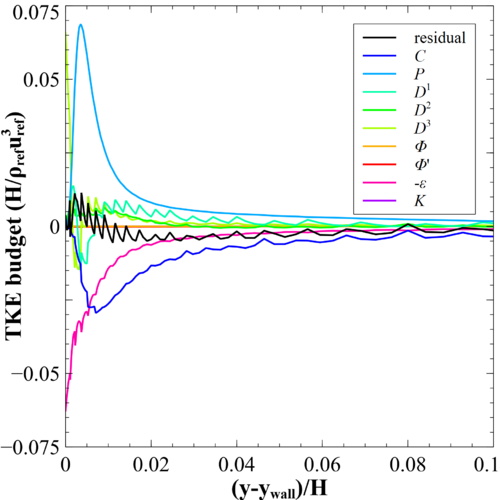Figure 10: HiFi-TURB-DLR rounded step, Reynolds-stress xx and TKE budgets at location $x/H=4$: convection $C$, production $P$, turbulent diffusion $D^{1}$, pressure diffusion $D^{2}$, viscous diffusion $D^{3}$, pressure strain $\Phi$, pressure dilatation $\Phi '$, dissipation $\varepsilon$and density fluctuation $K$.